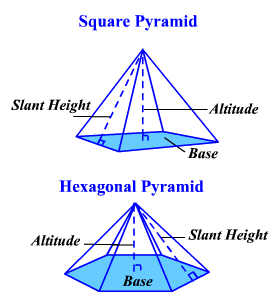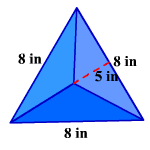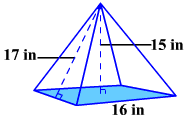Surface Area of a Pyramid

The lateral surface area of a regular pyramid is the sum of the areas of its lateral faces.

The total surface area of a regular pyramid is the sum of the areas of its lateral faces and its base.The general formula for the lateral surface area of a regular pyramid is $L.S.A.=\frac{1}{2}pl$ where $p$ represents the perimeter of the base and $l$ the slant height.

Example 1:

Find the lateral surface area of a regular pyramid with a triangular base if each edge of the base measures $8$ inches and the slant height is $5$ inches.The perimeter of the base is the sum of the sides.

$p=3\left(8\right)=24\text{\hspace{0.17em}}\text{\hspace{0.17em}}\text{inches}$

$L.S.A.=\frac{1}{2}\left(24\right)\left(5\right)=60\text{\hspace{0.17em}}\text{\hspace{0.17em}}{\text{inches}}^{2}$

The general formula for the total surface area of a regular pyramid is $T.S.A.=\frac{1}{2}pl+B$ where $p$ represents the perimeter of the base, $l$ the slant height and $B$ the area of the base.

Example 2:

Find the total surface area of a regular pyramid with a square base if each edge of the base measures $16$ inches, the slant height of a side is $17$ inches and the altitude is $15$ inches.The perimeter of the base is $4s$ since it is a square.

$p=4\left(16\right)=64\text{\hspace{0.17em}}\text{\hspace{0.17em}}\text{inches}$

The area of the base is ${s}^{2}$ .

$B={16}^{2}=256\text{\hspace{0.17em}}\text{\hspace{0.17em}}{\text{inches}}^{2}$

$\begin{array}{l}T.S.A.=\frac{1}{2}\left(64\right)\left(17\right)+256\\ \text{\hspace{0.17em}}\text{\hspace{0.17em}}\text{\hspace{0.17em}}\text{\hspace{0.17em}}\text{\hspace{0.17em}}\text{\hspace{0.17em}}\text{\hspace{0.17em}}\text{\hspace{0.17em}}\text{\hspace{0.17em}}\text{\hspace{0.17em}}\text{\hspace{0.17em}}\text{\hspace{0.17em}}\text{\hspace{0.17em}}\text{\hspace{0.17em}}\text{\hspace{0.17em}}=544+256\\ \text{\hspace{0.17em}}\text{\hspace{0.17em}}\text{\hspace{0.17em}}\text{\hspace{0.17em}}\text{\hspace{0.17em}}\text{\hspace{0.17em}}\text{\hspace{0.17em}}\text{\hspace{0.17em}}\text{\hspace{0.17em}}\text{\hspace{0.17em}}\text{\hspace{0.17em}}\text{\hspace{0.17em}}\text{\hspace{0.17em}}\text{\hspace{0.17em}}\text{\hspace{0.17em}}=800\text{\hspace{0.17em}}\text{\hspace{0.17em}}{\text{inches}}^{2}\end{array}$

There is no formula for a surface area of a non-regular pyramid since slant height is not defined.  To find the area, find the area of each face and the area of the base and add them.